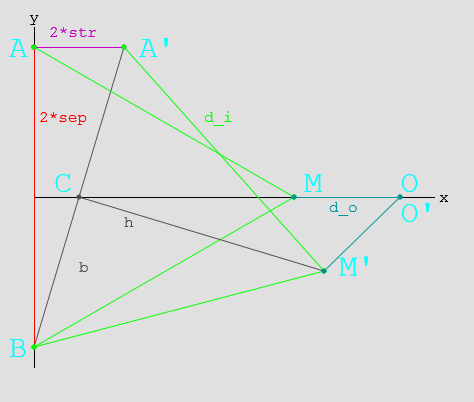In my previous post, I simulated some mechanical logic gates using a novel 3-bar linkage.
The dimensions of the linkage are critical to achieving correct operation;

Inputs $$\{ false\ false \}$$, $$\{ false\ true \}$$, $$\{ true\ false \}$$ must all produce the same output position.

For the simulations, an empirical approach was used. (fiddle with the values until it looks right)

This time, I’m going to attempt to find the exact values to use for a given stroke, separation distance and output position.Here, you can see the linkage layout for $$\{ false\ false \}$$ and $$\{ true\ false \}$$

# Mathematics

Ahead lurks tough mathematics (which may be unsuitable for liberal-arts majors)
If it’s hard to read, then sorry! It was hard to write!

## Parameters

• $$2 sep$$ Separation between inputs
• $$2 str$$ Stroke length $$false \to true$$
• $$d_i$$ Input link length
• $$d_o$$ Output link length

## Geometry

• $$A(x_a, y_a) = A(0, sep)$$ is the input position when $$A_{state}=false$$
• $$A'(x_a’, y_a’) = A'(2*str, sep)$$ is the input position when $$A_{state}=true$$
• $$B(x_b, y_b) = B(0,-sep)$$ is the input position (The system is symmetric, $$B’$$ does not need to be tested.)
• $$M(x_m, y_m)$$ is the middle position when $$A_{state}=false$$
• $$M'(x_m’, y_m’)$$ is the middle position when $$A_{state}=true$$
• $$O(x_o, y_o)$$ is the output position. ($$O \equiv O’$$ for the gate to function correctly.

## Solving

### Finding $$M$$:

$$M(x_m, y_m) = M( \sqrt{d_i^2 – sep^2}, 0)\\$$

### Finding $$O$$:

\begin{align} O(x_o, y_o) &= O( x_m + d_o, 0 )\\ & = O( \sqrt{d_i^2 – sep^2} + d_o, 0) \end{align}

### Finding $$M’$$:

• $$C'(x_c’, y_c’)$$ is the midpoint of $$BA’$$
• $$b$$ is the length of the line $$BC’$$
• $$h$$ is the length of the line $$C’M’$$
\begin{align} x_c’ &= (x_a’ + x_b)/2 \\ &= str\\ y_c’ &= (y_a’ + y_b)/2 \\ &= 0\\ b &= \sqrt{ sep^2 + str^2 }\\ h &= \sqrt{ d_i^2 – b^2 } && Because BC’ \bot C’M’\\ &= \sqrt{ d_i^2 – sep^2 – str^2 }\\ \end{align}\\

Using this, we can calculate M’:
\begin{align} \frac{h}{b} &= \frac{\sqrt{ d_i^2 – sep^2 – str^2 }}{\sqrt{ sep^2 + str^2 }} \\ &= \sqrt{\frac{ d_i^2 – sep^2 – str^2 }{ sep^2 + str^2 }} \\ &= \sqrt{\frac{d_i^2}{sep^2 + str^2} – 1}\\ \\ x_m’ &= x_c’\ – ( y_c’ – y_b)\frac{h}{b}\\ &= str – \sqrt{\frac{d_i^2}{sep^2 + str^2} – 1} \\ y_m’ &= y_c’ + ( x_c’ – x_b)\frac{h}{b}\\ &= str \sqrt{\frac{d_i^2}{sep^2 + str^2} – 1} \end{align}\\

### Finding $$O’$$:

\begin{align} x_o’ &= x_m’ + \sqrt{ d_o^2 – y_m’^2 }\\ &= ( str – \sqrt{\frac{ d_i^2 }{sep^2 + str^2} – 1}) + \sqrt{ d_o^2 – ( str \sqrt{\frac{d_i^2}{sep^2 + str^2} – 1} )^2 }\\ &= str – \sqrt{\frac{ d_i^2 }{sep^2 + str^2} – 1} + \sqrt{ d_o^2 – str^2 (\frac{d_i^2}{sep^2 + str^2} – 1) }\\ y_o’ &= 0 \end{align} \\

## And then:

Now that $$O$$ and $$O’$$ are found, and we know $$O \equiv O’$$…

### Finding the $$x_o’$$ equation in terms of $$d_o^2$$:

\begin{align} x_o’ &= x_m’ + \sqrt{ d_o^2 – y_m’^2 }\\ d_o^2 – y_m’^2 &= (x_o’ – x_m’)^2 \\ d_o^2 – str^2 (\frac{d_i^2}{sep^2 + str^2} – 1) &= (x_o’ – str + \sqrt{\frac{d_i^2}{sep^2 + str^2} – 1})^2 \\ d_o^2 &= \left( x_o’ – str + \sqrt{\frac{d_i^2}{sep^2 + str^2} – 1} \right)^2 + str^2 (\frac{d_i^2}{sep^2 + str^2} – 1) \\ \end{align}

### Substituting back into the $$x_o$$ equation:

\begin{align} x_o &= \sqrt{d_i^2 – sep^2} + d_o \\ x_o – \sqrt{d_i^2 – sep^2} &= d_o \\ ( x_o – \sqrt{d_i^2 – sep^2} )^2 &= d_o^2 \\ ( x_o – \sqrt{d_i^2 – sep^2} )^2 &= \left( x_o’ – str + \sqrt{\frac{d_i^2}{sep^2 + str^2} – 1} \right)^2 + str^2 (\frac{d_i^2}{sep^2 + str^2} – 1) \end{align}
Ugly, but workable?

# Example:

• $$sep = 50$$ Separation between inputs $$100 mm$$
• $$str = 20$$ Stroke length $$false \to true: 40 mm$$
• $$d_i = ?$$ Input link length
• $$d_o = ?$$ Output link length
• $$x_o = x_o’ = 70$$ Desired output position

\begin{align} ( x_o – \sqrt{d_i^2 – sep^2} )^2 &= \left( x_o’ – str + \sqrt{\frac{d_i^2}{sep^2 + str^2} – 1} \right)^2 + str^2 (\frac{d_i^2}{sep^2 + str^2} – 1) \\ ( 70 – \sqrt{d_i^2 – 50^2} )^2 &= \left( 70 – 20 + \sqrt{\frac{d_i^2}{50^2 + 20^2} – 1} \right)^2 + 20^2 (\frac{d_i^2}{50^2 + 20^2} – 1) \\ \end{align} \\
Pushed through Wolfram Alpha…
\begin{align} \\ d_i &= \sqrt{2900} \\ &= 53.852\\ \text{and}\\ d_o &= x_o – \sqrt{d_i^2 – sep^2} \\ &= 70 – \sqrt{ 2900 – 2500 } \\ &= 50 \end{align}

# Summary:

Phew!

Thusly, one can calculate the input and output linkage lengths for any logic gate.
These dimensions will work for an OR gate too, as the linkages are just reversed.

It’s irksome that I couldn’t work out a symbolic solution for the last equations, especially since $$d_i$$ in the example came out to such a neat value.

I might revisit this further in the future, and it’ll definitely come in handy when building something with these gates.